LEGACY CONTENT. If you are looking for Voteview.com, PLEASE CLICK HERE

This site is an archived version of Voteview.com archived from University of Georgia on May 23, 2017. This point-in-time capture includes all files publicly linked on Voteview.com at that time. We provide access to this content as a service to ensure that past users of Voteview.com have access to historical files. This content will remain online until at least January 1st, 2018. UCLA provides no warranty or guarantee of access to these files.

### 45-733 PROBABILITY AND STATISTICS I Notes #1A

First Lecture, 12 January 1999

#### Introduction

1. The theory of probability is concerned with the possible outcomes of an experiment.
2. Definition: An experiment is a process with at least two possible outcomes.
3. Definition: A Sample Space is the set S of all possible outcomes to an experiment.
4. For example, for the experiment, "toss one die", the set of outcomes is:
S = {1, 2, 3, 4, 5, 6}
For the experiment, "draw a card randomly from a deck of 52 standard playing cards", the set of outcomes is:
S = {Aª, Kª, Qª,..., 2ª, A©,..., 2©, A¨,..., 2¨, A§,..., 2§}
5. Definition: An Event is any subset of the sample space, S.
6. For example, the event, "even number" with respect to the experiment of tossing one die is:
E = {2, 4, 6}
With the probability of the Event being:
P(E) = 3/6 = 1/2
The event, "King" with respect to the experiment of drawing a card randomly from a deck of 52 standard playing cards, is:
E = {Kª, K©, K¨, K§}
With the probability of the Event being:
P(E) = 4/52 = 1/13
7. Definition: Probability is the assignment of numbers to indicate the chances than an event, E, in a sample space, S, will occur.
8. On what basis are these numbers assigned? There is no clear-cut answer to this question. In the simple case of a die, it seems intuitively obvious that each face should be assigned the number 1/6. We can give our intuition some support with the Frequency Method of Assigning Numbers. However, this method is far from solving our difficulties because of the way people use the words referring to probablility and its synonyms for one-shot events. For example: "The probability that the Vikings will win the Superbowl is better than two-thirds;" or "I only have a 50-50 chance of passing my math exam tomorrow." We can give these one-shot numbers some grounding by appealing to the Subjective Method of Assigning Numbers in which probabilities are taken to be measures of the degree of personal belief.

In any event, regardless of how the numbers are assigned, once the assignment occurs, then the rest is simply mathematical logic.

#### II. A Review of Some Simple Set Theory

1. Subsets: If A and B are events, then we write B Ì A if every element of B is also contained within A.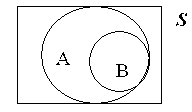For example, throw a die and let A be the event "all numbers greater than or equal to 2", and let B be the event "an even number appears"; that is,
A= {2, 3, 4, 5, 6} and B = {2, 4, 6}.
2. Union of Sets: The logical sum of two sets. AÈ B = {x|xÎ A or xÎ B or xÎ AÇ B}.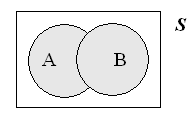3. Intersection or logical product of two sets: AÇ B = {x|xÎ A and xÎ B}.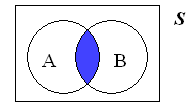4. Complement: The complement of a set A is all the elements of S not in A. That is, Ac = {x|xÏ A} and AÈ Ac = S and A Ç Ac = Æ, where Æ is the null or empty set.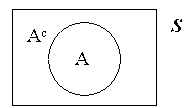We will use this a lot to solve problems. Oftentimes, it is easier to solve the probability for a complement than it is to solve the requested probability directly.
Example: The probability that a basketball player makes a freethrow on any particular try is .9. (We implicitly assume Independence here, we will define it later.) During a game she attempts 10 freethrows. What is the probability that she makes at least one basket? (Note that at least once is the union of the 10 sets: one or two or three or ....! To see this, assume she only makes two baskets and draw the Venn diagram!) To answer this question we use the complement which is that she misses all 10 freethrows. This is simply .110 so the answer is 1 - .110.
5. Mutually Exclusive Sets: A collection of sets such that no set in the collection has a non-empty intersection with any other set in the collection. For 3 sets, A, B, and C,
A Ç B = A Ç C = B Ç C = Æ.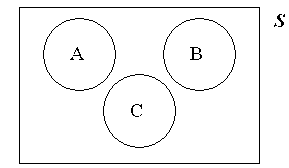6. Collectively Exhaustive Sets: A collection of sets such that the union of the collection is equal to the Sample Space. For 3 sets, A, B, and C: A È B È C = S.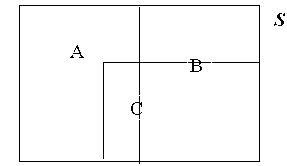7. K-Fold Partition of Sets: A collection of K sets that are both mutually exclusive and collectively exhaustive. We will use this concept when we work with Bayes Theorem.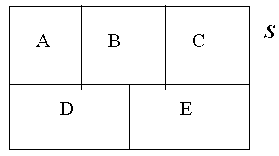#### III. The Axioms of Probability

1. The entire edifice of probability theory is generated from these 3 axioms:
axiom 1: All probabilities lie between 0 and 1.
axiom 2: The probability of the Sample Space is 1.
axiom 3: The probability of the union of mutually exclusive events is the sum of their separate probabilities.
2. The probability of the null set is 0 and the probability of an event is equal to 1 minus the probability of the complement of the event; that is,
P(Æ) = 0 = 1 - P(S), and
P(A) = 1 - P(Ac).
3. If A Ì B, then P(A) P(B). This is easily demonstrated with a simple Venn Diagram.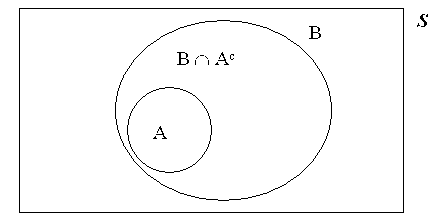The "trick" is to see that the set B can be written as the union of the mutually exclusive sets A and B Ç Ac. By applying axiom 3 it is easy to show the truth of the statement. Namely,
B = A È (B Ç Ac ), so from Axiom 3:
P(B) = P(A) + P(B Ç Ac ) P(A).
4. The Probability of the Union of two Events, A and B, is equal to the sum of their separate probabilities minus their intersection. That is,
P(A È B) = P(A) + P(B) - P(A Ç B)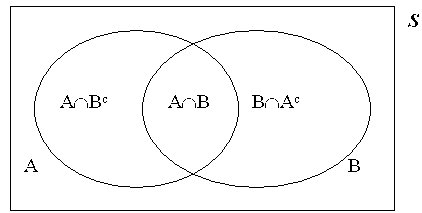The truth of this statement can be seen by using the same approach as in (18). The Union of A and B can be written as the union of the three mutually exclusive sets:
A Ç Bc; A Ç B; B Ç Ac.
Similarly, A can be written as the union of the two mutually exclusive sets:
A Ç Bc; and A Ç B.
And B can be written as the union of the two mutually exclusive sets:
A Ç B and B Ç Ac. The proof immediately follows. Namely;
P(A È B) = P(A Ç Bc) + P(A Ç B) + P(B Ç Ac)
P(A) + P(B) = P(A Ç Bc) + 2P(A Ç B) + P(B Ç Ac)
Hence: P(A È B) = P(A) + P(B) - P(A Ç B)
5. Problem: We have two Events A and B. If P(A)=.5 and P(B)=.6, what are the minimum and maximum values of the probability of A intersect B? Answer:
Using (19) above, (A È B) = .5 + .6 - P(A Ç B) 1,
which implies that P(A Ç B) .1, so that .1 is the minimum.
Using (18) above, if A Ì B, then P(A) = P(A Ç B) = .5, so that .5 is the maximum.

#### IV. Counting Methods

1. The basic idea of counting methods is simply to form the ratio of the number of elements in the Event, E, to the number of elements in the Sample Space, S. The ratio is the probability of E. That is:
P(E) = (number of elements in E)/(number of elements in S)
2. Simple examples of counting problems are in (6) above.
3. Problem: We roll 6 dice. What is the probability that each number appears exactly once? Answer: Clearly S contains 66 elements. The Event "Each number appears exactly once" has 6! elements. To see this, think about rolling one die at a time. You do not care which number comes up first. Now remove that number from the second die so it is five-sided and roll it: 6x5. Etc. (Note that when you are counting the number of elements in an event you are not doing probability!) Hence:
P(E) = 6!/66
4. People and Chairs. The number of permutations of n elements taken k at a time. The classic example is the number of ways that n people can be placed in k chairs (n k). The answer is:
n(n-1)(n-2)...(n-k+1) = n!/(n-k)! = Pkn
This is easy to see by doing the thought experiment of randomly assigning one person at a time to the chairs.
5. Boxes and Balls. Suppose we have 20 boxes and 15 balls. We toss the balls randomly into the boxes. What is the probability that when we are finished that no box contains more than one ball? Clearly the sample space has 2015 elements. To count the number of outcomes in the Event "no box contains more than one ball" do the following thought experiment. Imagine tossing the balls into the boxes one at a time. After you toss the first ball go and remove the box that it lands in. This leaves 19 boxes. Now toss the second ball and then remove the box that it lands in. Etc. Hence: 20x19x18... = 20!/5!
6. The Birthday Problem. What is the probability that in a group of n people that at least two people have the same birthday? (Caveats: just day and month; no twins; ignore Feb. 29). To get the answer, use the rule of the complement and find the probability that everyone has a different birthday. (This is like having 365 boxes and n balls!). Namely,
Let E = {At Least Two People Have the Same Birthday}. Hence
P(E) = 1 - P(Everyone has a Different Birthday). Using the Boxes and Balls Logic:
P(Everyone has a Different Birthday) = (365!/(365-n)!)/365n
By the Rule of the Complement the answer is: 1 - (365!/(365-n)!)/365n
With n = 23, P(E) = .507; when n= 30, P(E) = .706; and when n = 60, P(E) = .994.

7. Combinations (Binomial Coefficients). The classic example of a binomial coefficient is committee formation; viz., suppose we have n people and we want a committee of k people (n ³ k). How many different ways can we form the committee? Answer:
```
ænö
ç ÷
èkø
```
8. Note that:
```
ænö   æ n ö           ænö     ænö
ç ÷ = ç   ÷    and    ç ÷  =  ç ÷  =  1
èkø   èn-kø           ènø     è0ø
```
9. Example. Suppose a judge has 50 people from which to randomly choose a jury of 12 people. How many different juries could she form? Answer:
```
æ50ö     50!
ç  ÷ =  ------
è12ø    12!38!
```
Note that we do not care in what order the jurors sit in the jury box! We are only interested in the collection of 12 people. This is why the division by 12! is in the formula. If this were a simple people in chairs problem, the answer would be 50!/38!. To get the unique number of collections of 12 people we divide the 50!/38! by 12! because the 50!/38! includes all the permutations of each collection of 12 people.
10. Example. Suppose we have 30 people -- 20 women and 10 men. We have to randomly select 8 people to form a committee. What is the probability that we get exactly 4 women and exactly 4 men on the committee?
The event here is "4 women and 4 men". To get the probability of the event, we need to form the ratio of the number of elements in E to the number of elements in the sample space, S. Clearly, the number of elements in S is: [30 choose 8]. To get the number of elements in E think of the men and women as being in separate groups. If we randomly pick 4 women from the 20 women, [20 choose 4], and multiply it by the number of ways we could randomly pick 4 men from the 10 men, [10 choose 4], this is the number of elements in E. Hence:
```
æ20öæ10ö
ç  ÷ç  ÷
è 4øè 4ø
P(E) = ---------
æ30ö
ç  ÷
è 8ø
```
11. With reference to (6), what is the probability that our committee of 8 people will have at least 4 women?
This is a simple extension of (6). Note that this is the sum:
P[4 women, 4 men] + P[5 women, 3 men] + P[6 women, 2 men] + etc.
So the answer is:
```
æ20öæ 10 ö
ç  ÷ç    ÷
8 è jøè 8-jø
P(E) = å ---------
j=4  æ30ö
ç  ÷
è 8ø
```
12. Problem 2.33a-d MWS p. 44.
This is the same form as (5) only now our two groups are 4 organizers and 46 non-organizers from which we pick 3 winners (a committee of size 3). The Sample Space is: S = [50 choose 3] and the event for part (a) is
E= {the 3 winners are also organizers}.
```
æ4öæ46ö
ç ÷ç  ÷
è3øè 0ø
P(E) = ---------
æ50ö
ç  ÷
è 3ø
```
The answers for (b), (c), and (d) all have the same form as (a).
13. 6 Dice Problem ((23) in notes #1)
Think of the 6 dice in an urn. To count the number of elements in the event, "each number appears exactly once", randomly pick one die and make it the number one. This can be done [6 choose 1] ways since any one of the dice could be the number one. Now randomly pick one die from the 5 remaining to be the number two. This can be done [5 choose 1] ways. Etc. Answer:
```
æ6öæ5öæ4öæ3öæ2öæ1ö
ç ÷ç ÷ç ÷ç ÷ç ÷ç ÷
è1øè1øè1øè1øè1øè1ø
P(E) = --------------
66
```
14. Playing Card Problems. A standard deck of 52 playing cards consists of two kinds of objects: Denominations, 2, 3, 4, 5, 6, 7, 8, 9, 10, J, Q, K, A (in that order); and Suits, Spades (ª), Hearts (©), Diamonds (¨), Clubs (§). There are 13 denominations with 4 cards in each denomination; and there are 4 suits with 13 cards in each suit.

First Problem. Suppose we randomly select 4 cards. What is the probability that we get exactly one card in every suit? For Example: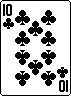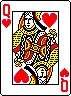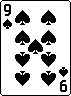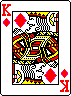This is just an elaboration of the men-women committee problem in (5). Only now we have four groups, not two. Clearly our sample space has [52 choose 4] elements. To get the probability we have to count the number of elements in the event "exactly one card in every suit".
The technique here is the same used above. Think of the 52 cards separated into the four suits (just as we separated the men and women). Now pick one card from each group of 13 cards. This is the number of elements in E. Answer:
```
æ13öæ13öæ13öæ13ö
ç  ÷ç  ÷ç  ÷ç  ÷
è 1øè 1øè 1øè 1ø
P(E) = --------------
æ52ö
ç  ÷
è 4ø
```
15. Suppose we randomly select 5 cards. What is the probability that we get 2 cards from one denomination and 3 cards from a second denomination? For example: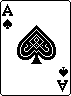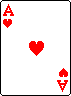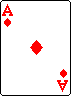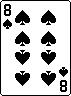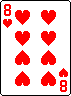Clearly the sample space has [52 choose 5] elements. To get the probability we have to count the number of elements in the event "2 cards from one denomination and 3 cards from a second denomination".
The technique here is more complicated than the previous problems. Here we have 13 denominations from which we choose 2. This is the number of pairs of denominations: A,K; 2,3; 4,J; A,8; 2,3; etc. However, note that for every pair of denominations, e.g., Aces and Kings, there are two ways you can get the event; viz., AAAKK and AAKKK respectively. Consequently, you have to take that into account: [2 choose 1]. Now you have one denomination designated as the one that will be the 3 cards, and one denomination designated as the one that will be the 2 cards. Now you draw 3 from the first and 2 from the second thereby counting all the possible elements in the event. Answer:
```
æ13öæ2öæ4öæ4ö
ç  ÷ç ÷ç ÷ç ÷
è 2øè1øè3øè2ø
P(E) = --------------
æ52ö
ç  ÷
è 5ø
```
16. Suppose we randomly select 5 cards. What is the probability that we get 2 cards from one denomination and 1 card each from three other denominations. For example: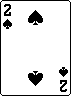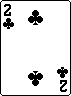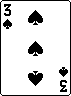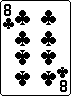Clearly the sample space has [52 choose 5] elements. To get the probability we have to count the number of elements in the event "2 cards from one denomination and 1 card each from three other denominations".
The technique here is similar to (11). To count the number of elements in E we first select 4 denominations, then we select one of the 4 denominations to be the one from which we draw 2 cards, then we draw the cards from each denomination. Answer:
```
æ13öæ4öæ4öæ4öæ4öæ4ö
ç  ÷ç ÷ç ÷ç ÷ç ÷ç ÷
è 4øè1øè2øè1øè1øè1ø
P(E) = ---------------
æ52ö
ç  ÷
è 5ø
```
17. Suppose we randomly select 5 cards. What is the probability that we get a sequence in one suit. For example,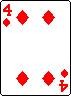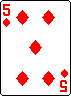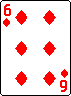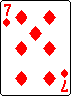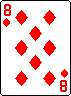(By definition, the order of the denominations is: 2, 3, 4, 5, 6, 7, 8, 9, 10, J, Q, K, A.)
Clearly the sample space has [52 choose 5] elements. To get the probability we have to count the number of elements in the event "sequence in one suit".
This is a disguised version of the men-women problem (5). Here the two groups are the 4 suits and the 9 possible sequences: 23456; 34567; 45678; etc. To count the number of elements in the event we simply draw one suit and draw one sequence. Answer:
```
æ4öæ9ö
ç ÷ç ÷
è1øè1ø
P(E) = ---------
æ52ö
ç  ÷
è 5ø
```
18. Suppose we randomly select 5 cards. What is the probability that we get a sequence.
This is the same as (13) only we do not care about the suits of the cards. As in (13), to count the number of elements in the event "sequence" we have to pick one of the 9 possible sequences but in doing so we are selecting 5 denominations in sequence. Consequently, after we pick the sequence we draw one card from each of the corresponding denominations. Answer:
```
æ9öæ4ö5
ç ÷ç ÷
è1øè1ø
P(E) = ---------
æ52ö
ç  ÷
è 5ø
```
19. Multinomial Coefficients. A Multinominal coefficient is simply the product of binomial coefficients. In can be thought of as multiple committee formation.

For example, suppose we have 40 people and we want to randomly divide them into committees of sizes 15, 15, and 10. How many ways can we do this? A common sense answer is first to randomly choose committee number one -- [40 choose 15] -- then committee number two -- [25 choose 15] -- and the remaining 10 people are the third committee: that is;
```
æ40öæ25öæ10ö     40!        25!       10!        40!
ç  ÷ç  ÷ç  ÷ = ------- *  ------- * ------ = ----------
è15øè15øè10ø    15!25!     15!10!    10!0!    15!15!10!
```
Note that this is the same as:
```
æ40öæ30öæ15ö     40!        30!       15!        40!
ç  ÷ç  ÷ç  ÷ = ------- *  ------- * ------ = ----------
è10øè15øè15ø    10!30!     15!15!    15!0!    10!15!15!
```
and we write it as:
```
æ   40   ö
ç        ÷
è15 15 10ø
```
20. The general form of this is:
```
æ       n       ö     æ n  öæn-n1öæn-n1-n2 ö
ç               ÷  =  ç    ÷ç    ÷ç       ÷ ...
èn1 n2 n3 ... nk ø     è n1 øè n2 øè   n3   ø
which is equal to:

n!
----------------
n1!n2!n3! ... nk!
```
21. Suppose, as in (15), we have 40 people -- 25 men and 15 women -- and we are forming committees of size 15, 15, and 10 respectively. What is the probability that we get exactly 5 women on each committee?
This is just an elaboration of (5). Clearly the number of elements in our sample space is the multinomial coefficient given in (15): [40 choose 15,15,10]
To count the number of elements in the event "exactly 5 women on each committee" we can use exactly the same technique as we used in (5) but now we do it for all three committees.
The number of ways the first committee could be formed is: [15 choose 5][25 choose 10].
For the second committee: [10 choose 5][15 choose 10].
The remaining 5 women and 5 men are the third committee.
Hence the answer is:
```
æ15öæ25öæ10öæ15öæ5öæ5ö
ç  ÷ç  ÷ç  ÷ç  ÷ç ÷ç ÷
è 5øè10øè 5øè10øè5øè5ø
P(E) = --------------------
æ   40   ö
ç        ÷
è15 15 10ø
```
Note that we can get the same answer by randomly dividing the 15 women up into three groups of 5 each, corresponding to the three committees; and randomly dividing the 25 men up into groups of 10, 10, and 5, corresponding to the three committees. This product of two multinomial coefficients is the number of elements in the event. Hence the answer is:
```
æ 15  öæ   25  ö
ç     ÷ç       ÷
è5 5 5øè10 10 5ø
P(E) = ---------------
æ   40   ö
ç        ÷
è15 15 10ø
```
22. Example 2.10, p. 39-40:
The book's answer for this problem is:
```
æ   16  ö
ç       ÷
è2 4 5 5ø
P(E) = ---------
æ   20  ö
ç       ÷
è6 4 5 5ø
```
However, we could have used the same reasoning as in (17). Namely, there are two kinds of laborers -- 4 from the ethnic group, and 16 others. To form the first job (a "committee" of size 6), we need all 4 members of the ethnic group and 2 from the others. Hence, there are:
[4 choose 4][16 choose 2] ways the first job requiring 6 laborers could have been randomly filled. Continuing with the same reasoning, for the second job there are [4 choose 0][14 choose 4] ways of choosing the laborers. Etc. Hence:
```
æ   16  ö    æ4öæ16öæ4öæ14öæ4öæ10öæ4öæ5ö   æ16öæ14öæ10öæ5ö
ç       ÷ =  ç ÷ç  ÷ç ÷ç  ÷ç ÷ç  ÷ç ÷ç ÷ = ç  ÷ç  ÷ç  ÷ç ÷
è2 4 5 5ø    è4øè 2øè0øè 4øè0øè 5øè0øè5ø   è 2øè 4øè 5øè5ø
```
Similarly:
```
æ   20  ö    æ20öæ14öæ10öæ5ö
ç       ÷ =  ç  ÷ç  ÷ç  ÷ç ÷
è6 4 5 5ø    è 6øè 4øè 5øè5ø
```
Hence the following is equivalent to the book's answer:
```
æ16öæ14öæ10öæ5ö
ç  ÷ç  ÷ç  ÷ç ÷
è 2øè 4øè 5øè5ø
P(E) = --------------
æ   20  ö
ç       ÷
è6 4 5 5ø
```
23. Example 2.10, p. 39-40 (Cont.):
Recall that the book's answer for this problem is:
```
æ   16  ö
ç       ÷
è2 4 5 5ø
P(E) = ---------
æ   20  ö
ç       ÷
è6 4 5 5ø
```
Another way to think about this problem is to first divide the 4 laborers from the specific ethnic group into the 4 jobs and multiply that by the the number of ways that the 16 other labors can be divided into the 4 jobs. Clearly, there are:
```
æ   4   ö
ç       ÷
è4 0 0 0ø
```
ways that the 4 labors can all be placed in the bad job. Hence:
```
æ   4   öæ   16  ö
ç       ÷ç       ÷
è4 0 0 0øè2 4 5 5ø
P(E) = -----------------
æ   20  ö
ç       ÷
è6 4 5 5ø
```